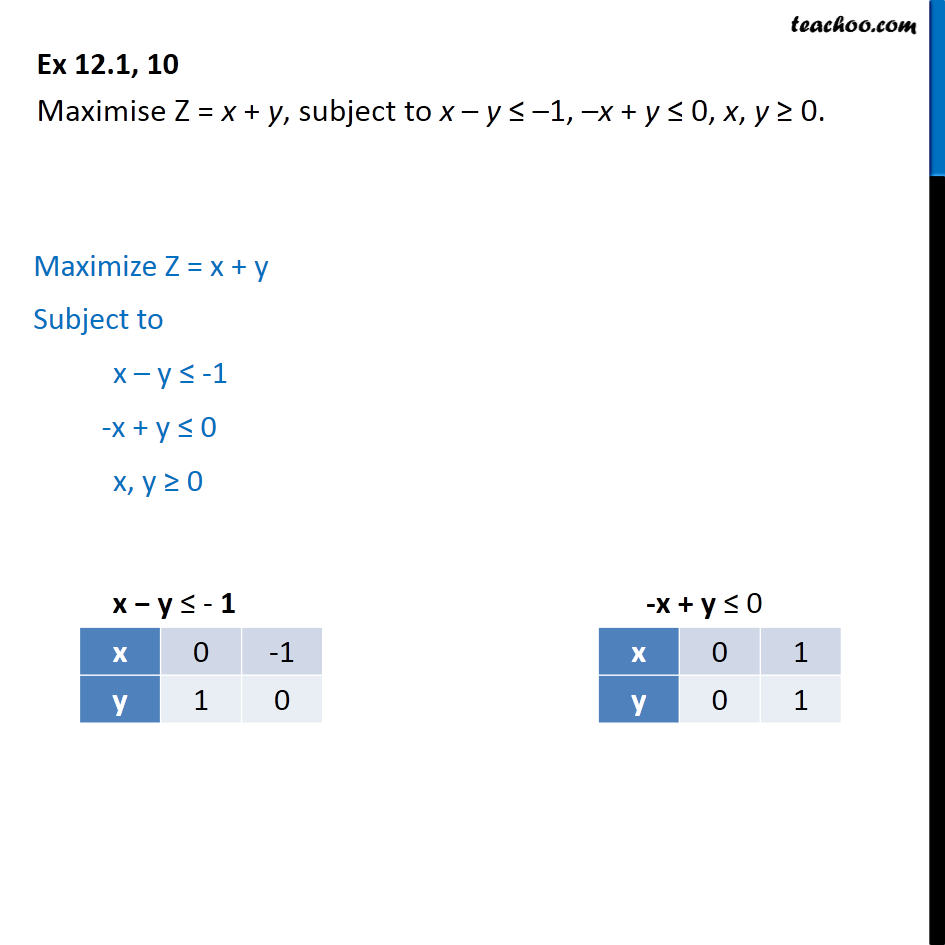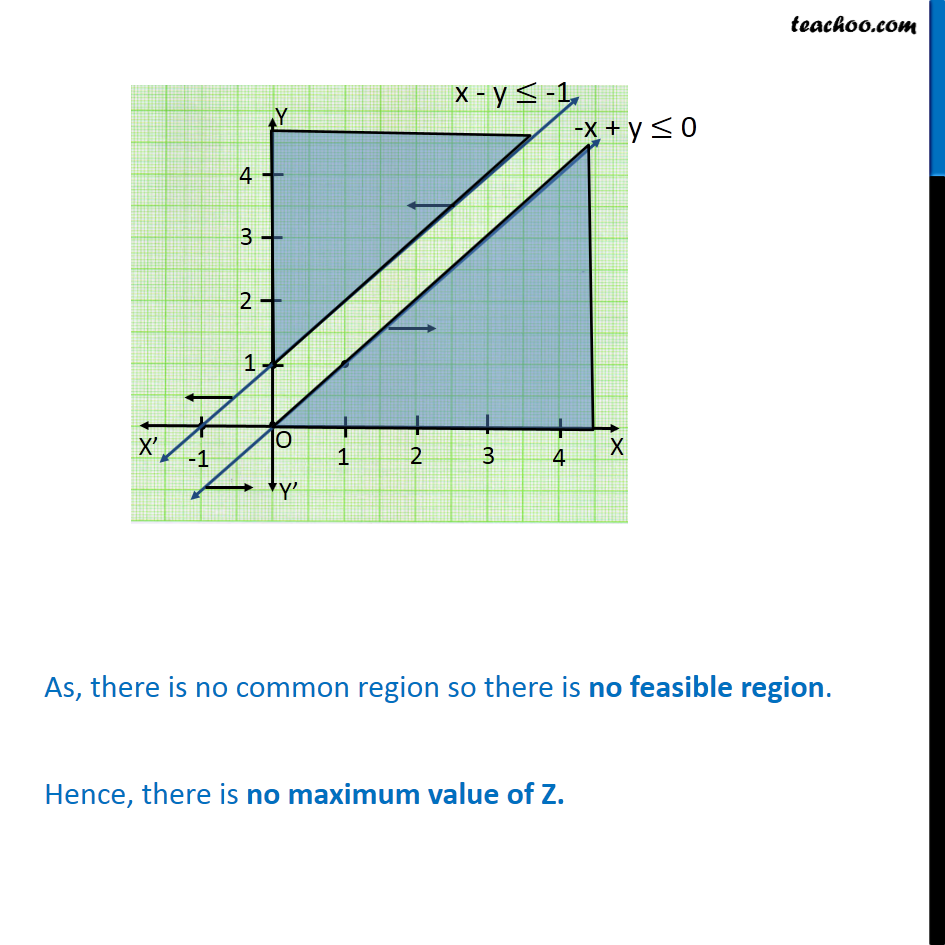1. Chapter 12 Class 12 Linear Programming
2. Serial order wise
3. Ex 12.1

Transcript

Ex 12.1, 10 - Chapter 2 Class 12 Linear Programming - NCERT Maximise Z = x + y, subject to x – y ≤ –1, –x + y ≤ 0, x, y ≥ 0. Plotting points For x – y ≤ -1 Points are (0, 1) and (-1, 0) For -x + y ≤ 0 Points are (0, 0) and (1, 1) Making graphs for both lines and shading the region. We notice that There is no common region ' Thus, there is no feasible region. Hence, there is no maximum value of Z

Ex 12.1